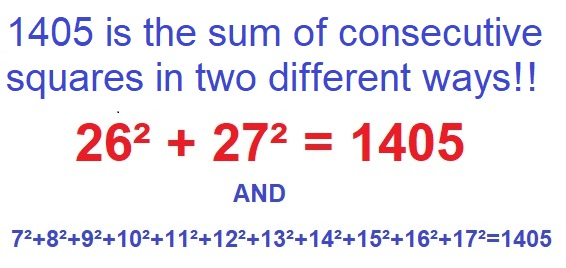1405 is the Sum of Squares

I knew that 1405 was the sum of two consecutive squares, but OEIS.org let me know that it was the sum of even more consecutive squares, ELEVEN to be exact!Because it is the sum of the 26th and the 27th squares, 1405 is also the 27th centered square number. Here are 1405 tiny squares illustrating that fact:Here’s more about the number 1405:

• 1405 is a composite number.
• Prime factorization: 1405 = 5 × 281.
• 1405 has no exponents greater than 1 in its prime factorization, so √1405 cannot be simplified.
• The exponents in the prime factorization are 1, and 1. Adding one to each exponent and multiplying we get (1 + 1)(1 + 1) = 2 × 2 = 4. Therefore 1405 has exactly 4 factors.
• The factors of 1405 are outlined with their factor pair partners in the graphic below.I’ve mentioned one of these before, but 1405 is the sum of TWO squares in TWO ways:
27² + 26² = 1405
37² + 6² = 1405

1405 is also the hypotenuse of FOUR Pythagorean triples:
53-1404-1405 calculated from 27² – 26², 2(27)(26), 27² + 26²
444-1333-1405 calculated from 2(37)(6), 37² – 6², 37² + 6²
800-1155-1405 which is 5 times (160-231-281)
843-1124-1405 which is (3-4-5) times 281

This site uses Akismet to reduce spam. Learn how your comment data is processed.